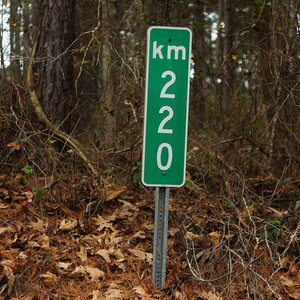# 10 most difficult units riddles

A list of ten (out of 19) units related questions that are the most often answered incorrectly
Which of the following length units is the largest?
meter
foot
ell
yard
One yard is 0,91 m; one foot is 0,3 m; ell was typically 60-70 cm.
Correct this question
0
Correct this question
0
Very interesting!
What unit is used to measure horses?
foot
hand
inch
yard
Horses and ponies are measured by hands (1 hand equals 4 inches).
Correct this question
0
Correct this question
0
Very interesting!
Play and see more questions